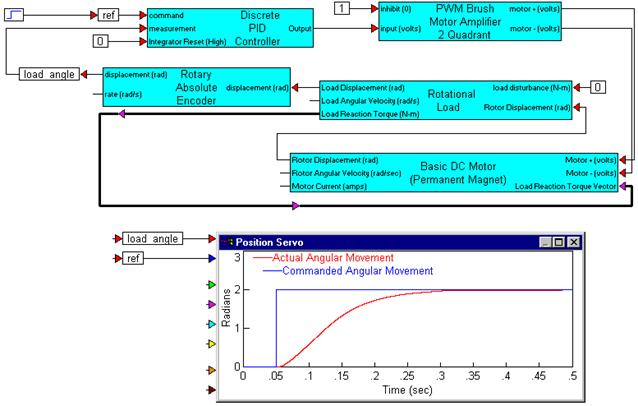### Basic (Permanent Magnet) DC Motor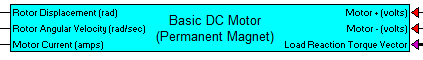Category: Motors

The Basic (Permanent Magnet) DC Motor block is derived from the linear system model of a simple DC permanent magnet machine. It is assumed that the back EMF is equal to the torque constant, and that the torque current relation is indeed constant over the rotation of the motor. Input requires a DC voltage and a load reaction torque vector if external mechanical load is used.

Mechanical dynamics include typical parameters, such as rotor shaft inertia and viscous friction. In addition, nonlinear dissipative factors, including Coulomb friction and stiction models are provided. The Basic (Permanent Magnet) DC Motor block can operate stand-alone to produce output displacement or velocity of the motor alone, or when a Rotational Load block is connected, combined dynamic response. When connected to the Rotational Load block, dynamic parameters are reflected back and combined with the motor dynamics by the linkage ratio. The linkage ratio is specified in the Rotational Load block. This creates proper dynamic motion of the combined motor-load connection. To connect Basic (Permanent Magnet) DC Motor block to the Rotational Load block, the rotor displacement and load reaction vector connections from each block must be wired together.

Rotor shaft position, velocity and motor current are provided for sensor connections in monitoring and feedback applications.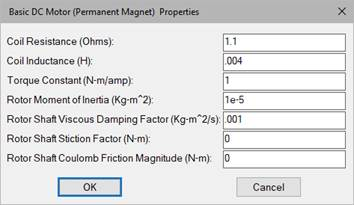Coil Resistance: Indicates the line-to-line coil resistance in ohms.

Coil Inductance: Indicates the line-to-line coil inductance in henries.

Torque Constant: Indicates the specified motor torque constant in N-m/Amp.

Rotor Moment of Inertia: Indicates the moment of inertia of the rotor with respect to the axis of rotation in kg-m2.

Rotor Shaft Viscous Damping Factor: Indicates the factor that linearly relates viscous damping force to angular velocity. This option is normally not specified by the motor manufacturer, but can be determined experimentally. Units are in kg-m2/s.

Rotor Shaft Stiction Factor: Allows specification of a stiction force value or break-away torque. This option is normally not specified by the motor manufacturer, but can be obtained experimentally. Units are N-m.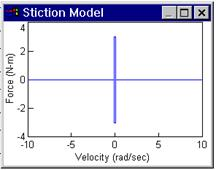Rotor Shaft Coulomb Friction Magnitude: Allows specification of constant directional dissipative force (Coulomb model) in units of N-m.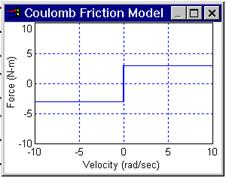#### Example

Diagram name: Basic DC Motor

Location: Examples > eMotor > Brush DC

The following simulation example demonstrates a voltage-controlled servo loop for positioning a geared load (0.5 ratio) to multiply torque. The load is spring loaded by setting a torsional spring constant in the Rotational Load block. A Rotary Encoder block is coupled to the load and the measurement is fed back to a PID compensator for voltage control of the motor. A power amplifier of unity voltage gain with sufficient power gain to drive the motor is assumed. The motor is commanded to move 2 rad in the positive direction.